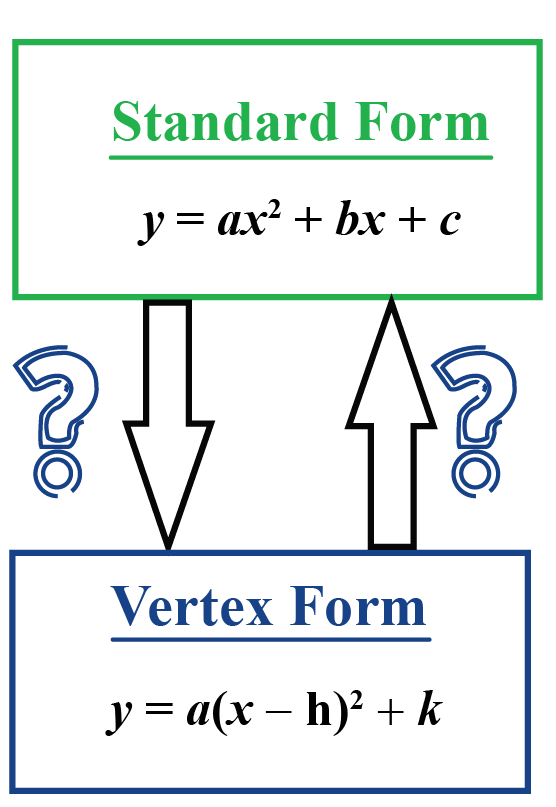# Standard form to vertex form

Standard form to vertex form

In this mini-lesson, we will explore the process of converting standard form to vertex form and vice-versa.

The standard form of a parabola is $$y=ax^2+bx+c$$.

The vertex form of a parabola is $$y=a(x-h)^2+k$$.

Here, the vertex form has a square in it.

So to convert the standard to vertex form we need to complete the square.

Let's learn about the following in detail:

• Standard form to vertex form
• Vertex form to standard form## Lesson Plan

 1 How to Convert Standard Form To Vertex Form? 2 Important Notes on Standard Form to Vertex Form 3 Tips and Tricks on Standard Form to Vertex Form 4 Solved Examples 5 Interactive Questions

## How to Convert Standard Form To Vertex Form?

### Standard Form

The standard form of a parabola is:

 $$y=ax^2+bx+c$$

Here, $$a, b,$$ and $$c$$ are real numbers (constants) where $$a \neq 0$$.

$$x$$ and $$y$$ are variables where $$(x,y)$$ represents a point on the parabola.

### Vertex Form

The vertex form of a parabola is:

 $$y=a(x-h)^2+k$$

Here, $$a,h,$$ and $$k$$ are real numbers where $$a\neq 0$$.

$$x$$ and $$y$$ are variables where $$(x,y)$$ represents a point on the parabola.

More Important Topics
Numbers
Algebra
Geometry
Measurement
Money
Data
Trigonometry
Calculus
More Important Topics
Numbers
Algebra
Geometry
Measurement
Money
Data
Trigonometry
Calculus